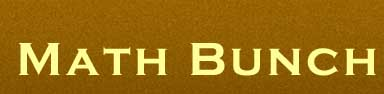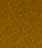Feed Back Membership Ask Question Free Download Help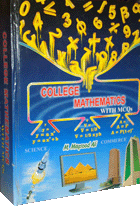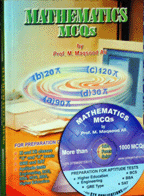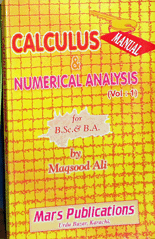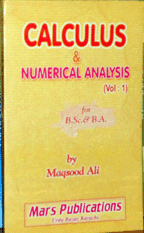COLLEGE MATHEMTICS WITH MCQs Topic Arrangements >>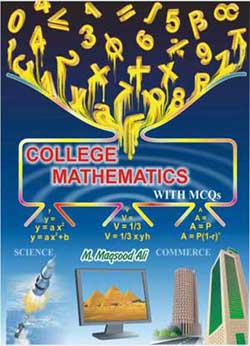The author wished that title of the book must show the application of mathematics and he succeeded to sketch the title of the book which expresses that how mathematics forms the equations using number, letters and symbols.

This book has got manifold qualities but some of them are pinpointed here under.

• This book has been printed in two colours . The words of each topic are shown in colours to which a topic depends so that the book is made interesting and understandable.

• The book has been written in such a way as if students are being taught in the class room. The method of “ Write as we Talk ” has been adopted in this book through which the difficult problems and definitions can be understood easily and quickly. The explanations of the topics is understandable easily.

• Ample use of diagrams, in two colours, for the sake of understanding easily.

• Maximum methods have been used to solve a problem.

• Usually it is difficult to differentiate among the Similar Topics but it has been made easy through their comparison in solved examples in detail. Similar topics have been chalked out, compared and differentiated in two separate columns with explanation , definition and solved examples.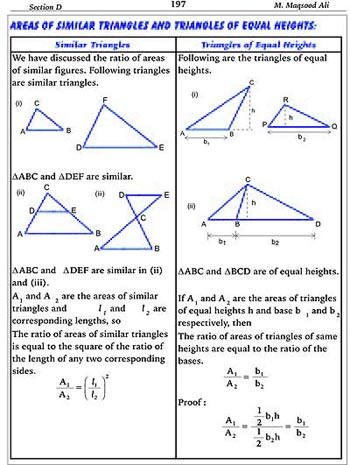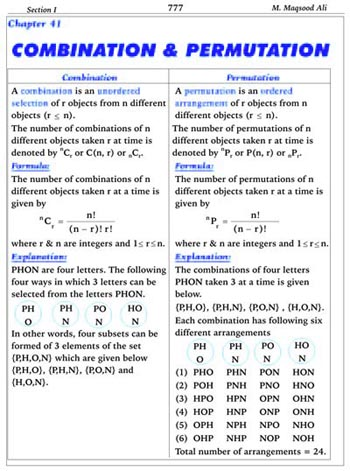• The diagrams have been frequently used to make the reader understand the topics and examples.

• Beside curve sketching , it has also been made clear in the book that how a curve is changed into other quickly , when a constant added, subtracted, multiplied or divided in a function or in an independent variable.

• As graphs have got key position in mathematics , so techniques of drawing graphs have been emphasized. It is also considered that students may understand the applications of graphs in examples and unsolved problems.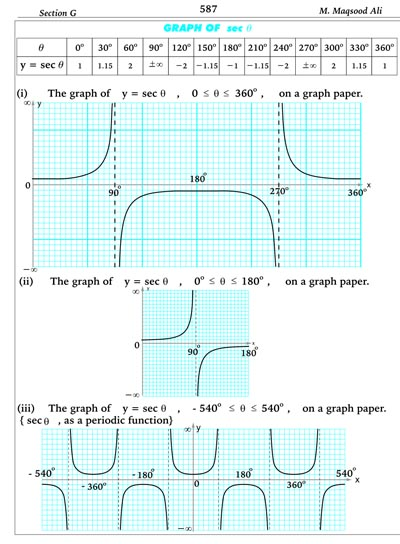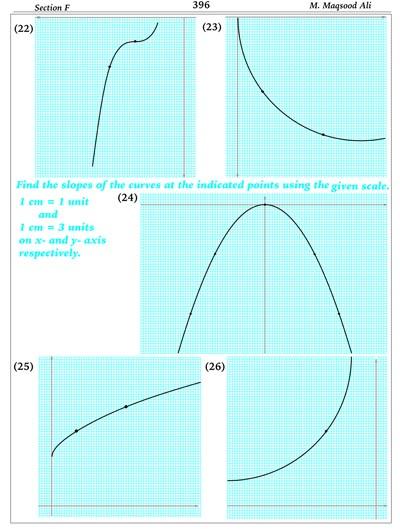• Every topic is further illustrated by solved examples after being defined and explained, so that student may attain expertise in the topic.

• MCQs serve as a quick mean to assess the knowledge of students these days. So MCQs in this book will help the students in self assessment.

• Unsolved problems in each exercise will be a source of further practice and to understand the applications of the topic .

• In order to make the students understand, practice and grasp the each topic has been divided and arranged in the following:
1- Definition
2- Explanation
3- Diagrams
4- Formulas
5- Shortcuts
6- Solved Examples
7- Unsolved Problems
8- MCQs

• This book has managed Top Arrangements.@ Math Bunch. All rights reserved. Powered By UiTech